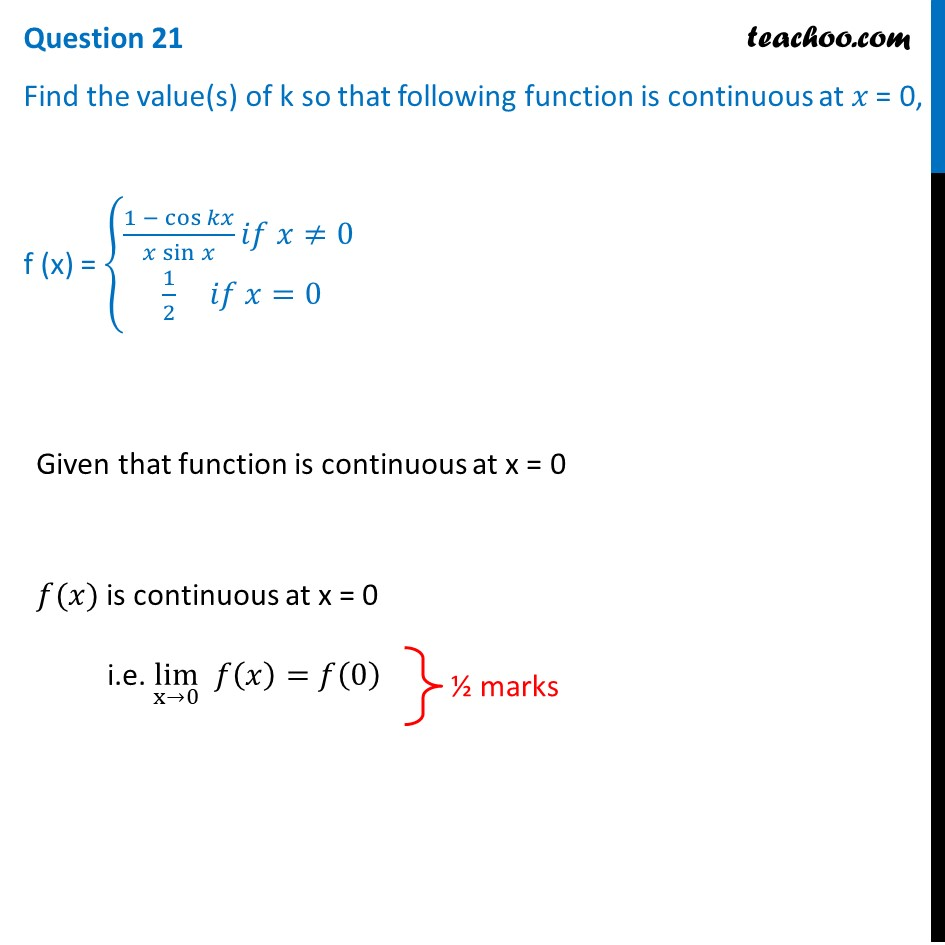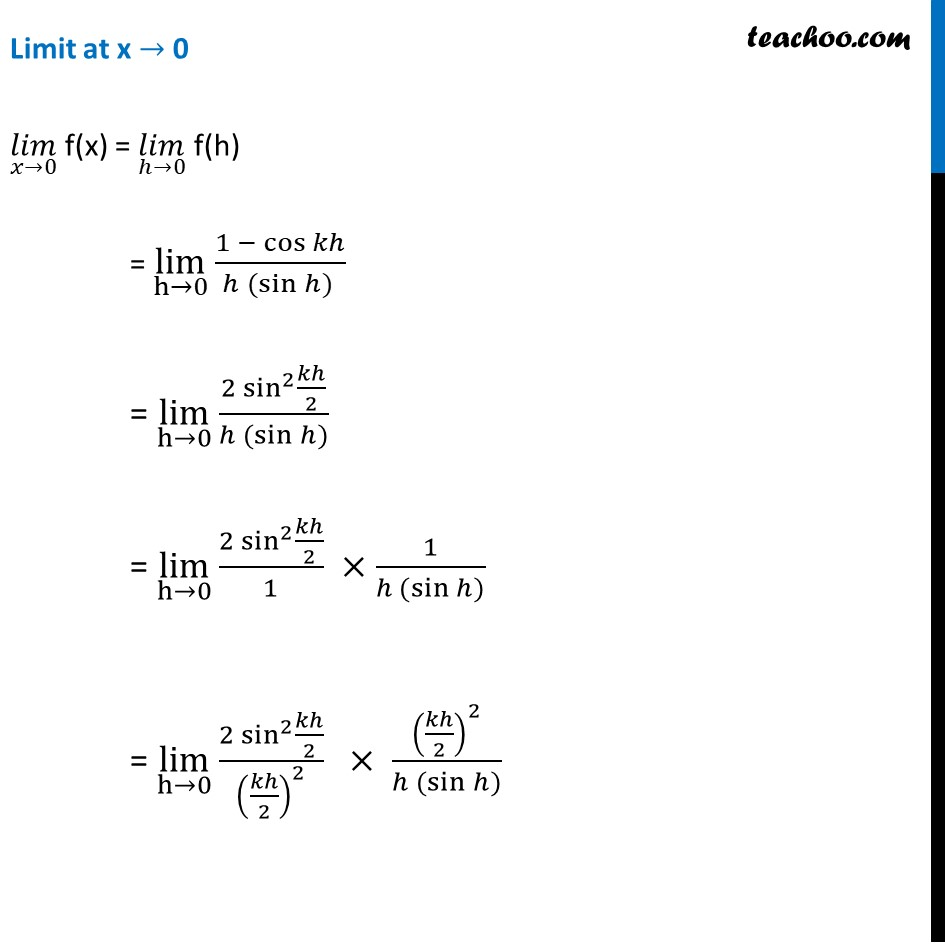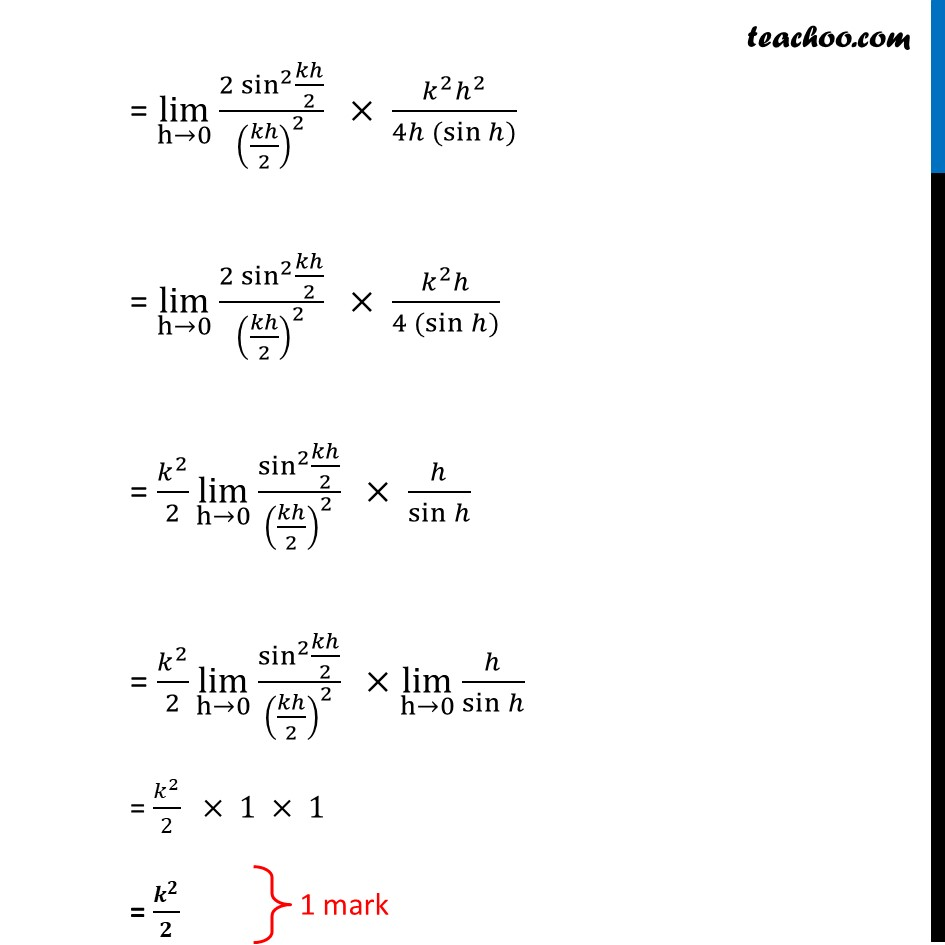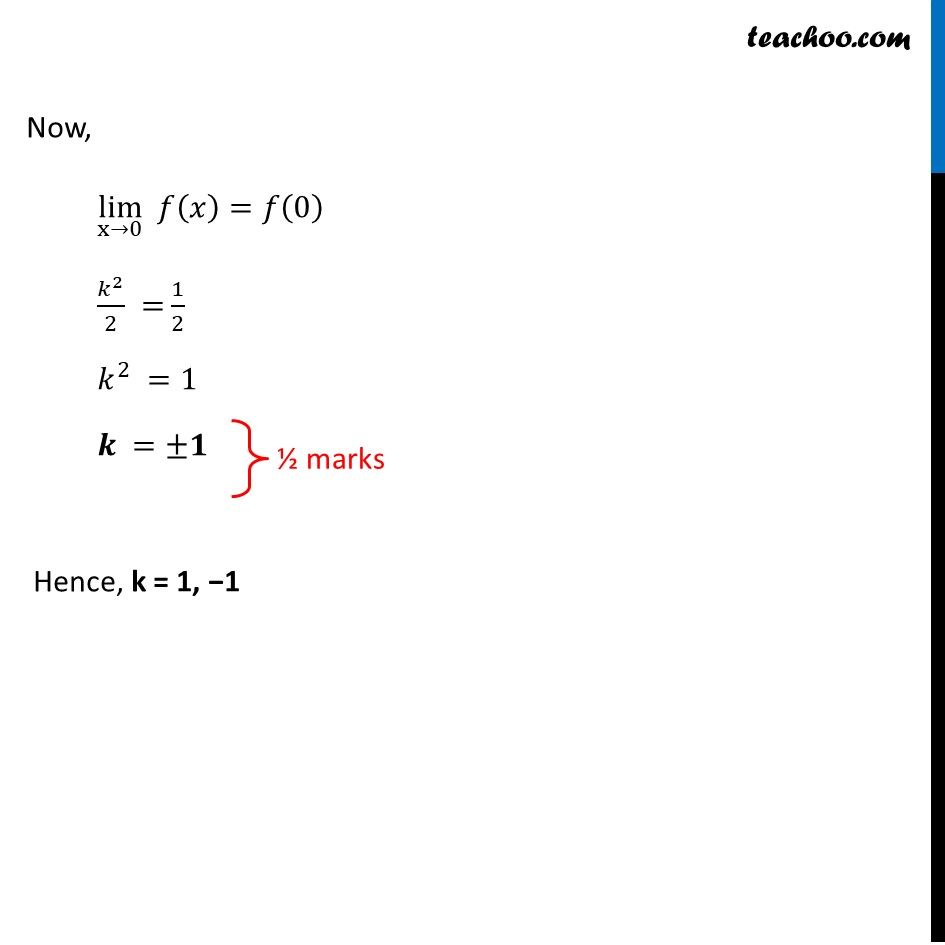## Find the value(s) of k so that the following function is continuous at 𝑥 = 0 f (x) = { 1- cos ⁡kx / x sin⁡x  if x≠0 1/2 if x=01. Class 12
2. Solutions of Sample Papers and Past Year Papers - for Class 12 Boards
3. CBSE Class 12 Sample Paper for 2021 Boards

Transcript

Question 21 Find the value(s) of k so that following function is continuous at 𝑥 = 0, f (x) = {█((1 − cos⁡𝑘𝑥)/(𝑥 sin⁡𝑥 ) 𝑖𝑓 𝑥≠0@ 1/2 𝑖𝑓 𝑥=0)┤ Given that function is continuous at x = 0 𝑓(𝑥) is continuous at x = 0 i.e. lim┬(x→0) 𝑓(𝑥)=𝑓(0) Limit at x → 0 (𝑙𝑖𝑚)┬(𝑥→0) f(x) = (𝑙𝑖𝑚)┬(ℎ→0) f(h) = lim┬(h→0) (1 − cos⁡𝑘ℎ)/(ℎ (sin⁡ℎ) ) = lim┬(h→0) (2 sin^2⁡〖𝑘ℎ/2〗)/(ℎ (sin⁡ℎ)) = lim┬(h→0) (2 sin^2⁡〖𝑘ℎ/2〗)/1 ×1/(ℎ (sin⁡ℎ)) = lim┬(h→0) (2 sin^2⁡〖𝑘ℎ/2〗)/(𝑘ℎ/2)^2 × (𝑘ℎ/2)^2/(ℎ (sin⁡ℎ)) = lim┬(h→0) (2 sin^2⁡〖𝑘ℎ/2〗)/(𝑘ℎ/2)^2 × (𝑘^2 ℎ^2)/(4ℎ (sin⁡ℎ)) = lim┬(h→0) (2 sin^2⁡〖𝑘ℎ/2〗)/(𝑘ℎ/2)^2 × (𝑘^2 ℎ)/(4 (sin⁡ℎ)) = 𝑘^2/2 lim┬(h→0) sin^2⁡〖𝑘ℎ/2〗/(𝑘ℎ/2)^2 × ℎ/sin⁡ℎ = 𝑘^2/2 lim┬(h→0) sin^2⁡〖𝑘ℎ/2〗/(𝑘ℎ/2)^2 ×lim┬(h→0) ℎ/sin⁡ℎ = 𝑘^2/2 × 1 × 1 = 𝒌^𝟐/𝟐 Now, lim┬(x→0) 𝑓(𝑥)=𝑓(0) 𝑘^2/2 = 1/2 𝑘^2 =1 𝒌 =±𝟏 Hence, k = 1, −1

CBSE Class 12 Sample Paper for 2021 Boards

Class 12
Solutions of Sample Papers and Past Year Papers - for Class 12 Boards

About the AuthorDavneet Singh
Davneet Singh is a graduate from Indian Institute of Technology, Kanpur. He has been teaching from the past 9 years. He provides courses for Maths and Science at Teachoo.